Courses

# Test: Static & Dynamic Force Analysis - 1

## 10 Questions MCQ Test Topicwise Question Bank for Mechanical Engineering | Test: Static & Dynamic Force Analysis - 1

Description
This mock test of Test: Static & Dynamic Force Analysis - 1 for Mechanical Engineering helps you for every Mechanical Engineering entrance exam. This contains 10 Multiple Choice Questions for Mechanical Engineering Test: Static & Dynamic Force Analysis - 1 (mcq) to study with solutions a complete question bank. The solved questions answers in this Test: Static & Dynamic Force Analysis - 1 quiz give you a good mix of easy questions and tough questions. Mechanical Engineering students definitely take this Test: Static & Dynamic Force Analysis - 1 exercise for a better result in the exam. You can find other Test: Static & Dynamic Force Analysis - 1 extra questions, long questions & short questions for Mechanical Engineering on EduRev as well by searching above.
QUESTION: 1

### Klein’s construction is a graphical method of determining

Solution:

Klein’s construction for reciprocating engine mechanism:
(i) has a simpler construction for getting quadrilateral similar to the diagram of mechanism for reciprocating engine.
(ii) is applicable when the crank has uniform angular velocity.
(iii) is used to determine the linear velocity and acceleration of piston.

QUESTION: 2

Solution:
QUESTION: 3

### The velocity of the piston of reciprocating engine is equal to ... where, ω = angular of crank, θ = angle turned by crank from IDC, r - radius of crank, n = ratio of length of connecting rod to crank radius

Solution: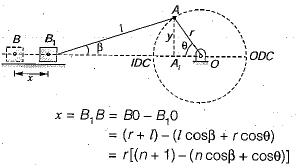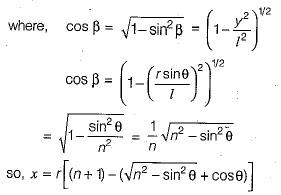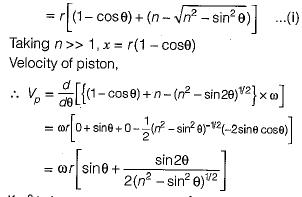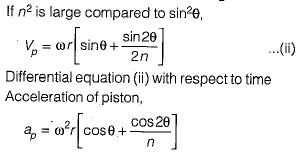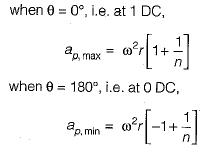At θ = 180°, when the direction of motion is reversed,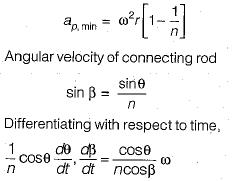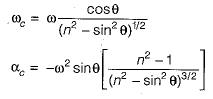QUESTION: 4

The displacement of the piston of a reciprocating enigne is equal to ....

(All symbols have their usual mearing)

Solution:
QUESTION: 5

The angular velocity of hte connecting rod of a reciprocating engine is equal to

Solution:
QUESTION: 6

The angular acceleration of the connecting rod of a reciprocating engine is equal to ....

(All symbols have their usual meaning)

Solution:
QUESTION: 7

A slider crank mechanism is shown in the given figure.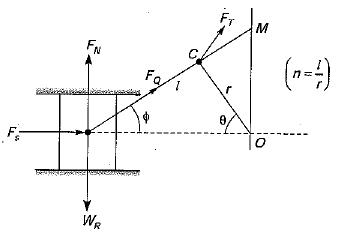Which of the following expressions stand for crank effort?

1.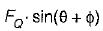2.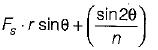3.  Fs . OM

4. FT.r

Select the correct answer using the codes given below

Solution:

Crank effort: Force is exerted on the crank pin as a result of the force on the piston. Crank effort is the net effort (force) applied at the crank pin perpendicular to the crank which gives the required turning moment on the crank shaft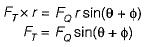QUESTION: 8

In the figure given below, when is the absolute velocity of end B of the coupler equal to the absolute velocity of the end A of the coupler?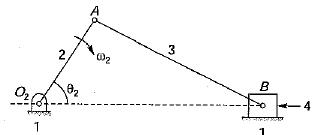Solution: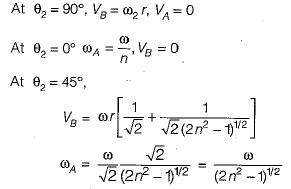QUESTION: 9

A plate is held by a chain AB through hinge joints. The plate is pulled with a force Tas shown in figure. The tension in the chain is given by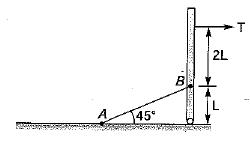Solution: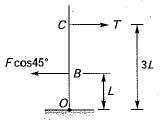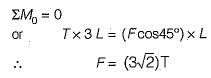QUESTION: 10

A pair of action and reaction forces acting on a body are known as

Solution: